## Characteristics of Regulated Power Supply:

The quality of power supply depends on different factors such as its load voltage, load current, voltage regulation, source regulation, output impedance, ripple rejection etc. Some of the characteristics of regulated power supply are discussed below.

1. Load Regulation: The load regulation, abbreviated LR (also called the load effect), is the change in regulated output voltage when the load current changes from minimum to maximum value i.e.,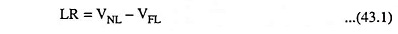where VNL is load voltage at no load and VFL is the load voltage at full load. In this equation VNL occurs when the load resistance is infinite (i.e., out terminals are open circuited) and VFL occurs when the load resistance is of the minimum value where voltage regulation is lost.

Load regulation is usually expressed in percentage and is given as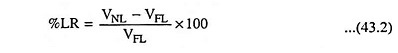For an ideal power supply output voltage should be independent of dc load current and the percentage load regulation should be equal to zero.

2. Minimum Load Resistance: Another characteristics of regulated power supply is load resistance, at which a power supply delivers its full-load rated current at rated voltage is referred to as a minimum load resistance, RL (min).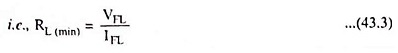The value of full-load current IFL should never increase beyond that mentioned in the data sheet of the power supply. For instance, if a data sheet specifies that the power supply will provide an output voltage of 24 V at a maximum rated current of 0.2 A, then minimum load resistance that can he connected across the supply is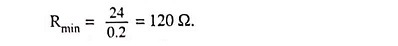If any attempt is made to decrease the value of load resistance RL below this value, the rated dc output voltage will not be available.

3. Source or Line Regulation: The input line voltage has a nominal value of 230 V but in practice, there are considerable variations in ac supply mains voltage. Since this ac supply mains voltage is the input to the ordinary power supply, the filtered output of the bridge rectifier is almost directly proportional to the ac mains voltage. Filtered output of the bridge rectifier is the input to the voltage regulating device.

Another way of specifying the quality of a regulated power supply is by its source regulation (also called the source effect or line regulation) abbreviated as SR. The source regulation, SR is defined as the change in regulated output voltage for a specified range of line voltage, typically 230 V ± 10 per cent. The defining equation is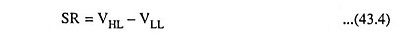where VHL is output voltage with high input ac line voltage and VLL is output voltage with low input ac line voltage.

The percentage of source regulation is given as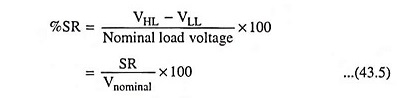The output voltage under specific operating conditions is known as the nominal load (or output) voltage.

4. Output Impedance. A regulated power supply is a very stiff dc voltage source. This means that the output resistance is very small (in milliohms). Even though the external load resistance is varied, almost no change is seen in the load voltage. An ideal voltage source has an output impedance of zero. Modern regulated power supplies approach ideal voltage sources.

5. Ripple Rejection: Voltage regulators stabilize the output voltage against variations in input voltage. Ripple is equivalent to a periodic variation in the input voltage. Thus, a voltage regulator attenuates the ripple that comes in with the unregulated input voltage. Since a voltage regulator uses negative feedback, the distortion is reduced by the same factor as the gain. Ripple rejection is a measure of a power supply’s ability to reject ripple voltages and is usually expressed in decibels.

Scroll to Top